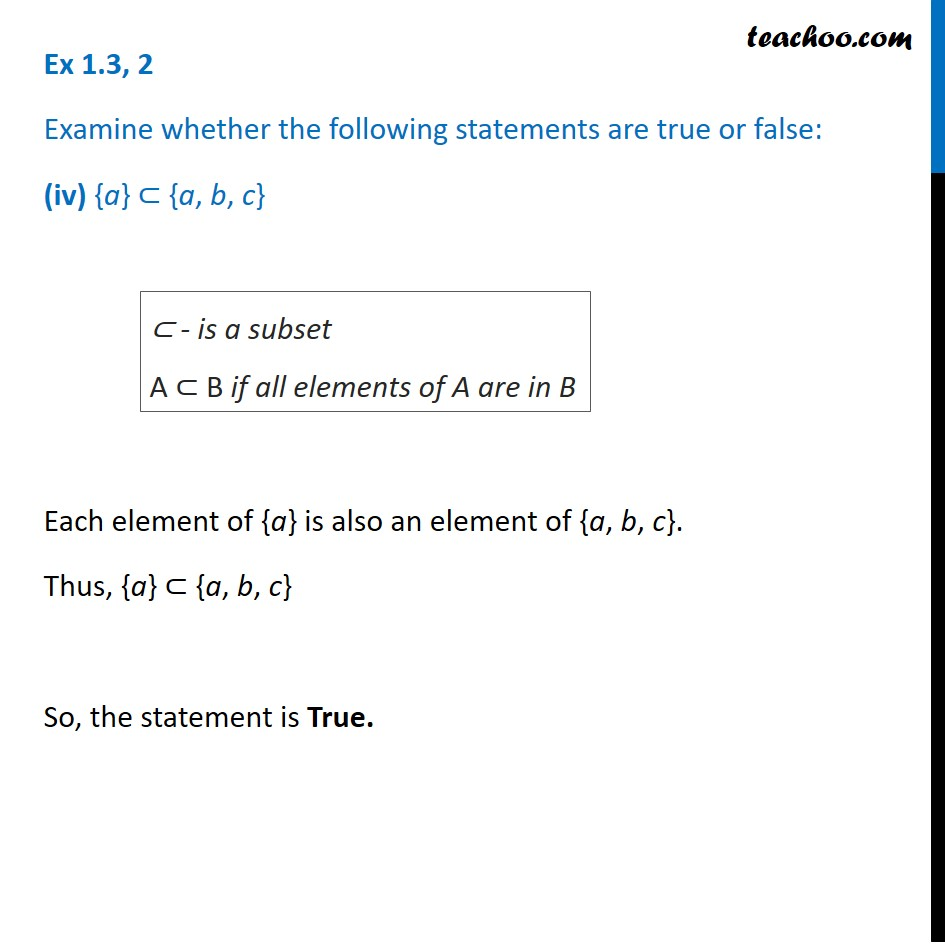1. Chapter 1 Class 11 Sets (Term 1)
2. Serial order wise
3. Ex 1.3

Transcript

Ex 1.3, 2 Examine whether the following statements are true or false: (iv) {a} ⊂ {a, b, c} Each element of {a} is also an element of {a, b, c}. Thus, {a} ⊂ {a, b, c} So, the statement is True. ⊂ - is a subset A ⊂ B if all elements of A are in B